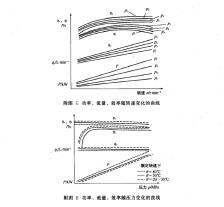Created on：2021-06-16 09:46

# Data processing and expression of experimental method for hydraulic gear pump

④ Experimental data processing and result expression

a. Data processing. Using the test data and the following calculation formula, the relevant performance indexes of the tested pump are calculated.

Volumetric efficiency:

ηv=V2,e/V2,e=(qv2,e/ne)/(qv2,i/ni) ×100%

Total efficiency:

ηt=(p2,eqv2,e-p1,eqv1,e)/(2πneT1) ×100%

Output hydraulic power in kW:

P2,h=(p2,eqv2,e)/60000×100%

Input mechanical power in kW:

P1m=（2πneT1）/60000

Where qv2, I -- output flow under no-load pressure, L / min;

Qv2, e -- output flow at test pressure, L / min;

Qv1, e -- input flow at test pressure, L / min;

NE -- rotational speed under test pressure, R / min;

Ni -- rotational speed under no-load pressure, R / min;

V2, e -- displacement at test pressure, ml / R;

V2, I -- no load displacement, ml / R;

P2, e -- output test pressure, kPa;

P1, e -- input pressure, which is positive when it is greater than atmospheric pressure and negative when it is less than atmospheric pressure, kPa;

T1 -- input torque, n · M.

b. The results were expressed. The test report shall include test data and relevant characteristic curves. Examples of characteristic curves are shown in figures g and H. The test report shall also provide the information of test personnel, equipment, working conditions and basic characteristics of the tested pump.Home    Article    Data processing and expression of experimental method for hydraulic gear pump